×#### Thank you for registering.

One of our academic counsellors will contact you within 1 working day.

Click to Chat

1800-1023-196

+91-120-4616500

CART 0

• 0

MY CART (5)

Use Coupon: CART20 and get 20% off on all online Study Material

ITEM
DETAILS
MRP
DISCOUNT
FINAL PRICE
Total Price: Rs.

There are no items in this cart.
Continue ShoppingWorking Rule to Calculate AreaArea under curves is an extremely important topic of mathematics syllabus of IIT JEE. Questions can be asked on computation of area of any kind of curve and hence students are advised to have mastery in curve tracing. If a person is able to draw a curve correctly, then it becomes easy to reach at the accurate solution.

In this section, we shall discuss some of the ways of calculating area in case of standard curves followed by working rules of calculating area.

(i) To determine the area enclosed between the curves

(a) y = f(x), x = a and x = b,

(b) x = f(y), y = a and y = b.Case (a) The area bounded by the curve y = f(x), x-axis and the ordinates x = a and x = b (where b > a) is given by the formula

Case (b) The area bounded by the curve x = f(y), y-axis and the ordinates y = a and y = b (where b > a) is given by the formula

(ii) To find the area bounded by the straight lines x = a, x = b (where a < b) and the curves y = f(x) and y = g(x), where f(x) < g(x) is given by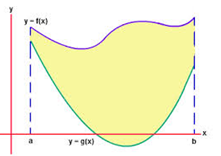Similarly, the area bounded by the straight lines y = a, y = b (where a < b) and the curves x = f(y) and x = g(y), where f(y) < g(y) is given by

(iii) To find the area bounded by the curves y = φ(x) and y = Ψ(x)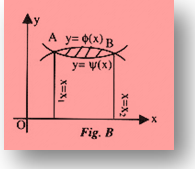If the roots of the intersection are x = x1 and x = x2, then the required area is given by

where x1 < x2 and x1 and x2 are the roots of the equation f(x) = g(x).

Similarly, if two curves x = f(y) and x = g(y) intersect, the required bounded area is given by

here x1 < x2 and x1 and x2 are the roots of the equation f(y) = g(y).

(iv) Area between the curve y = f(x) and y-axis: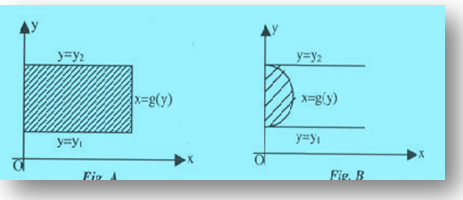To obtain the area between the curve and the y-axis, the function must be written in y. (see figure A and B) i.e. y = f(x) must be inverted to x = g(y) (where g(x) = f -1(x)) and the integral to be evaluated is A=

Similarly the area bounded between the y-axis & the curves y = f(x) and y = g(x) can be determined. In general, to find the area of the region one must draw the curve and locate the region. The limits and sign of different definite integral are determined accordingly.

(v) If the curve lies completely above the x-axis, then the area is positive but when it lies completely below the x-axis then the area is negative; however, we have the convention to consider the magnitude only.

(vi) If the curve lies on both the sides of the x-axis i.e. above the x-axis as well as below the x-axis, then calculate both areas separately and add their moduli to get the total area.In general if the curve y = f(x) crosses the x-axis n times when x varies from a to b, then the areas between y = f(x), the x-axis and the lines x = a and x = b is given by A = |A1| + |A2| +……+ |An|.

If the curve is symmetrical about the x-axis, or the y-axis, or both, then calculate the area of one symmetrical part and multiply it by the number of symmetrical parts to get the whole area.

Watch this Video for more reference

Illustration 1:

Find the area bounded by y = x |sin x| and the x-axis between x = 0, x = 2π.

Solution:

We can write the given function as

y = x sin x, when sin x ≥ 0 i.e. 0 ≤ x ≤ π

= -x sin x, when sin x < 0 i.e. π < x ≤ π

We first construct the graph to get a fair idea of the required region,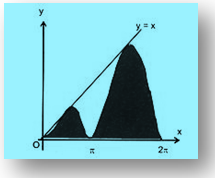Hence the required area A is_________________________________________________________________________________

Illustration 2:

Find the area bounded by the curve |x| + y = 1 and the x-axis.

Solution:

The given curve is |x| + y = 1 …… (1) which can be written as

x + y = 1, when x > 0

and –x + y = 1, when x < 0.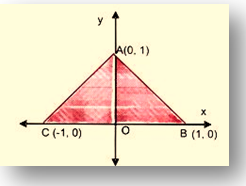The required area = area (CAOC) + area (OABO)= 1 sq. unit.

Note:

This question can also be solved directly as y = 1 – |x| is an even function. Hence graph of y = 1 – |x| is symmetrical about the y-axis. Thus the required area =

____________________________________________________________________________________

Illustration 3:

Calculate the area bounded by the curve y = x(3 – x)2, the x-axis and the ordinates of the maximum and minimum points of the curve.

Solution:

The given curve is y = x(3 – x)2. Now for points of maxima or minima, we have dy/dx = 0

⇒ (3 – x)2 – 2x(3 – x) = 0

⇒ (3 – x)(3 – x – 2x) = 0          ∴ x = 1, 3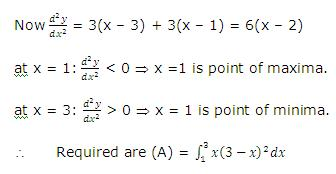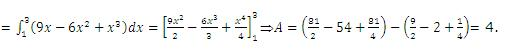_____________________________________________________________________________________

Illustration 4:

Find the area between the curves y = ex ln x and y = ln x /ex.

Solution:

The two curves intersect where ex ln x = ln x /ex.

⇒ (ex – 1/ex)ln x = 0

⇒ x = 1/e or x = 1 (ln x is not defined for x = –1/e)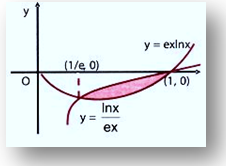At x = 1/e or ex = 1, we have ln x = -1, y = -1

⇒ (1/e, –1) is one point of intersection and at x = 1, ln 1 = 0, y = 0

⇒ (1, 0) is the other common point of the curves.

The required area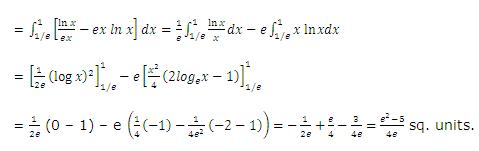_______________________________________________________________________________________

Illustration 5:

Calculate the area included between the lines x = 2, y = 2 and x + y = 5.

Solution:

The given lines are
x = 2                    ………… (i)
y = 2                    ………… (ii)
x + y = 5               ………… (iii)The curves are plotted in the figure given above and we are required to find the shaded area

For point Q put y = 2 in (iii) we get x = 3

Required area = Area ΔPQR

= (5x – x2/2 - 2x), where x varies from 2 to 3.

= 15 – 9/2 – 6 – 10 + 4/2 + 4

= 21 – 41/2 = 1/2 sq. units.

_________________________________________________________________________________

Illustration 6:

Find the area included between the curves y = sin-1x, y = cos-1x and the x-axis.

Solution:

Clearly we have to calculate the area of the shaded region OPBO. The point P of intersection of = y sin-1x and y = cos-1 x. Hence its coordinate are (1/√2,π/4)Put x = sin θ

⇒ dx = cosθ dθ so that

x = 0 ⇒ θ = 0

x = 1/√2 ⇒ θ = π/4,

x = 1 ⇒ θ = π/2 ⇒ The required area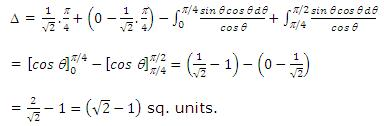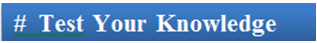Q1: The area bounded by the curves y = |x| – 1 and y = -|x| + 1 is

(a) 1

(b) 2

(c) 2√2

(d) 4

Q2: The area bounded by the curves y2 = 4ax and x2 = 4by is

(a) 16ab/3

(b) 16a2/b

(c) 16a/3b

(d) 16b/3a

Q3: What is the area enclosed between the curves y = f(x), x = a and x = b

(a)

(b)

(c

(d)

Q4: Area bounded by the straight lines y = a, y = b (where a < b) and the curves x = f(y) and x = g(y), where f(y) < g(y) is given by

(a)

(b)

(c)

(d) Can’t say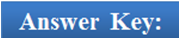Q1(b), Q2(a), Q3(a), Q4(a)

Related Resources

You might wish to refer Solved examples on area under curves.

For getting an idea of the type of questions asked, refer the previous year papers.

To read more, Buy study materials of Application of Integration comprising study notes, revision notes, video lectures, previous year solved questions etc. Also browse for more study materials on Mathematics here.### Course Features

• 731 Video Lectures
• Revision Notes
• Previous Year Papers
• Mind Map
• Study Planner
• NCERT Solutions
• Discussion Forum
• Test paper with Video Solution